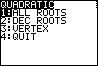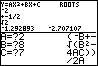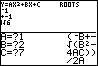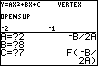## Overview

Solves quadratic equations. It gives an exact answer in simplified form for both real and imaginary roots. If there are real roots, it can give a decimal approximation. It also gives information, such as the vertex, about the parabola descibed by the equation such as the vertex.

## InstructionsAs in this example for 2x^2+8x+7=0 A=2, B=8, and C=7. The solution from the program is -2+-(1/2)*sqrt(2). All fractions are in lowest terms and as much as possible is taken out from under the square root. The decimal approximations also displayed. As you can see, if imaginary roots are required, the program will display an i in the correct place and display no real roots. The vertex portion of the program returns the vertex of the equation y=Ax^2+Bx+C.